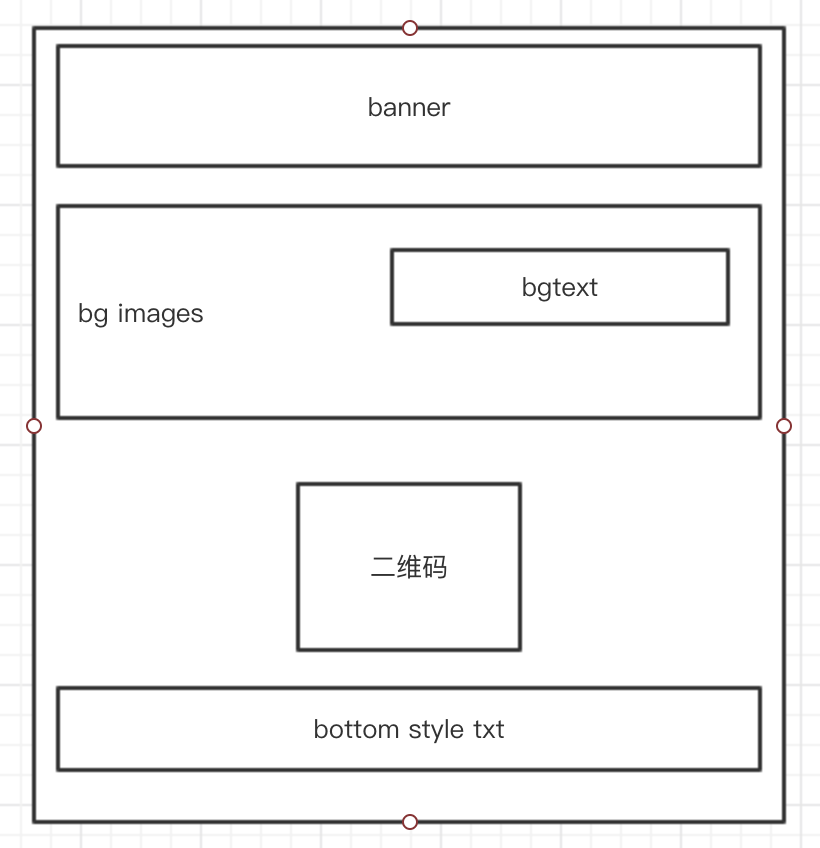# # canvas 踩坑记

## # 前言## # 踩坑过程

### # 图片位置计算

``````function loadImageSync(src) {
return new Promise(resolve => {
let img = new Image()
resolve(img)
}
img.src = src
})
}
``````

``````// 假设img_src1和img_src2是后台获取到的
let { img_src1, img_src2 } = this.data
let img1 = null
.then(img => {
img1 = img
ctx.drawImage(img1, 0, 0)
})
.then(img => {
ctx.drawImage(img, 0, img1.height)
})
``````

### # 文本换行

``````function textAutoLine(text, canvas, initX, initY, lineHeight) {
let ctx = canvas.getContext('2d')
// 等同于css的font
ctx.font = 'bold 18px Microsoft Yahei'
// 初始化一行字体的宽度为0
let lineWidth = 0
let canvasWidth = canvas.width
// 初始化截取位置为0
let lastSubStrIndex = 0
for (let i = 0; i < text.length; i++) {
// 循环计算每个字的宽度 并累加到行宽上
lineWidth += ctx.measureText(text[i]).width
// 判断行宽是否到达一个临界值 也就是： 容器宽 - 文字第一个字的位置 - 临界值（可自定义调整）
if (lineWidth > canvasWidth - (initX + 40)) {
// 一行达到临界，开始绘制，并且重置行宽，另起一行，开始绘制下一行
ctx.fillText(text.substring(lastSubStrIndex, i), initX, initY)
initY += lineHeight
lineWidth = 0
lastSubStrIndex = i
}
if (i === text.length - 1) {
// 第二行字 再做偏移
ctx.fillText(text.substring(lastSubStrIndex, i + 1), initX + 40, initY)
}
}
}
``````

### # 绘制 dom

``````function drawDomToImg(dom) {
let domData = `<svg xmlns="http://www.w3.org/2000/svg" width="500" height="200">
<foreignObject width="100%" height="100%">
<div xmlns="http://www.w3.org/1999/xhtml">\${dom}</div>
</foreignObject></svg>`
let DOMURL = window.URL || window.webkitURL || window
let svg = new Blob([domData], { type: 'image/svg+xml;charset=utf-8' })
let src = DOMURL.createObjectURL(svg)
}
``````

### # 图片跨域

``````function loadImageSync(src) {
return new Promise(resolve => {
let img = new Image()
// 加入这句
img.setAttribute('crossOrigin', 'Anonymous')